# A new method for drawing Algebraic Surfaces

Richard Morris (rich@singsurf.org)
University of Liverpool

July, 1992

This paper was presented at the Fifth IMA confrence on the Mathematics of Surfaces at Edinbrugh University, 1992 and appears in

Design and Applications of Curves and Surfaces, Ed R.B. Fisher, Clarendon Press, Oxford, 1994

# Introduction

In this paper we describe a program for drawing algebraic surfaces in . Strictly speaking it draws the zero sets of implicit polynomials . The program produces a model of the surface which can be viewed using a general rendering package. The program has been specially designed to draw singular surfaces accurately and find all the components of the surface.

There are many programs already existing which do this to a limited extent. Programs to draw surfaces of the type z=f(x,y) have been around for a number of years. CAD systems can draw specific types of algebraic surfaces but not normally singular ones. There are a few existing programs which draw general algebraic surfaces. Ray tracers  can be used to produce beautiful pictures of algebraic surface but they are slow and only gives the view from one direction. Other programs such the contour tracer written by the Geometry Supercomputer Project , produce good results but do not pay mush attention to singularities and may give topologically incorrect results.

A hybrid method has been used in the algorithm using recursive sub-division, Bernstein polynomials and a little differential geometry. The division of the surface into cells also plays an important part in the algorithm.

Thanks must go to R. Martin who introduced me to A. Geisow's method  for drawing algebraic curves in the plane which is based on Bernstein polynomials, D. Marsh for useful conversations and an overview of possible techniques, C. Gibson for doubting whether it was possible and hence providing the motivation and finally all those in the Liverpool University Pure Mathematics Department who produced many practical examples to test the routine on.

The pictures produced in this paper have been produced using an implementation of the algorithm running on a Silicon Graphics Iris 4D-GT graphics workstation provided by the S.E.R.C. under a computer science initiative grant. The models were displayed using the geomview package written at the Geometry Center, University of Minnesota.

# Figures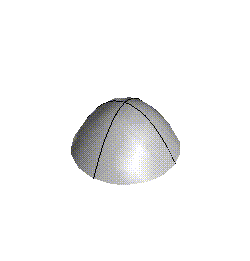Figure 1: Two smooth surfaces showing curves along which the partial derivatives vanish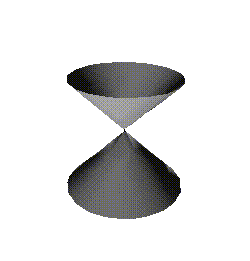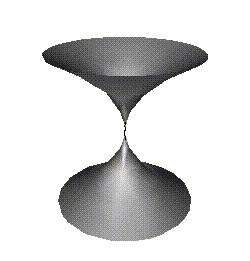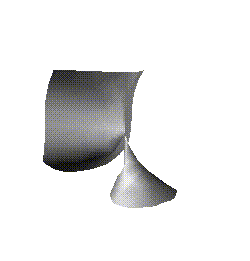Figure 2: Zero contours of some of the standard germs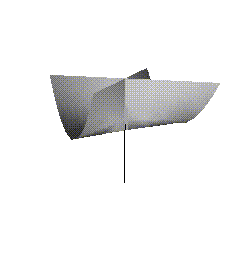Figure 3: A cross-cap and a triple point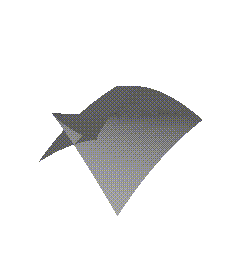Figure 4: A swallowtail surface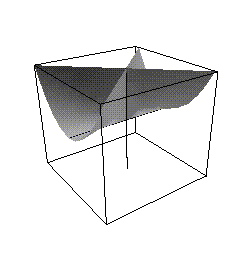Figure 5: Dividing a cross-cap into cells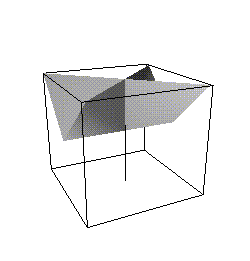Figure 6: approximating the cross-cap of Fig. 5 by a set of facets and linesFigure 7: Finding solutions on an edge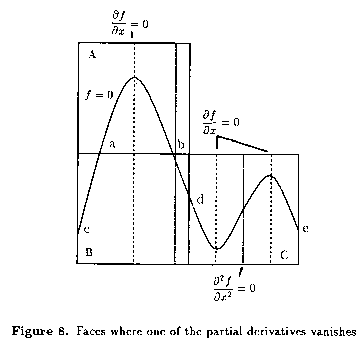Figure 8: Faces where one of the partial derivatives vanishes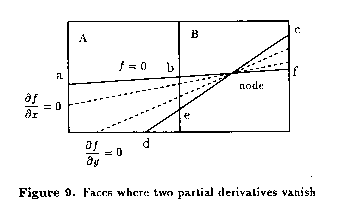Figure 9: Faces where two partial derivatives vanish

Back to My Papers